# Introduction to Complex Analysis

• Difficulty Level : Hard
• Last Updated : 06 Mar, 2022

Complex analysis is a branch of mathematics that deals with complex numbers, their functions, and their calculus. In simple terms, complex analysis is an extension of the calculus of real numbers to the complex domain. We will extend the notions of continuity, derivatives, and integrals, familiar from calculus to the case of complex functions of a complex variable. In doing so we will come across analytic functions, which form the centerpiece of this introduction. In fact, to a large extent complex analysis is the study of analytic functions.

The basic ingredient of complex analysis is an analytic function, or that we know so well in calculus as a differentiable function. Any complex number z can be thought of as a point in a plane (x,y), so z = x+iy, where i=√-1.  In a similar fashion, any complex function of a complex variable z can be separated into two functions, as in,  f(z)=u(z)+iv(z), or, f(x,y)=u(x,y)+iv(x,y). Clearly, such functions depend on two independent variables and have two separable functions, so plotting the function would need a four-dimensional space, which is difficult to imagine.

Of course, the first starting point of the calculus of complex functions is to start with continuity of the function and then slowly move into the differentiability in the complex domain.

### Continuity

We start with a rather trivial case of a complex-valued function. Suppose that f is a complex-valued function of a real variable. That means that if x is a real number, f(x) is a complex number, which can be decomposed into its real and imaginary parts:  f(x) = u(x)+i v(x), where u and v are real-valued functions of a real variable; that is, the objects you are familiar with from real calculus. We say that f is continuous at x0 if u and v are continuous at x0.

Continuity of a complex-valued function of a complex variable is a non-trivial property. A function is said to be continuous at a point zo if it approaches the value f(zo) when z approaches zo. Since z lives in a complex plane (also known as the Argand plane), it can approach the point from multiple directions. Hence we need to refine our definition to meet the new needs.

Formally, we say that f(z) is continuous at zo if, for any ε>0, there exists a δ>0, such that |f(z)-f(zo)|<ε for all |z-zo|<δ. So whenever z is inside a disk of radius δ centered at zo (called the neighborhood of zo) and the function approaches the value of f(zo).

### Analyticity

Once we have established the notions of continuity of complex functions, we can dive into the differentiation of complex functions. While discussing the differentiation of complex functions, we will see that such functions need to satisfy an important criterion, called the Cauchy-Riemann equations. If an analogy helps, we know that for the derivative of a real function to existing finitely at a given point, the function should be continuous at the point. A similar criterion for the complex functions is the Cauchy-Riemann equations. We shall soon see the proof in the following discussion.

A complex-valued function of a real variable can be readily differentiated, as f'(x)=u'(x)+iv'(x), where f(x) is the complex-valued function of a real variable x. In other words, we extend the operation of differentiation complex-linearly. There is nothing novel here.

However, the situation changes when f becomes a function of a complex variable z. We know that a complex variable z itself is actually a pair of two real variables x and y. We plot z as a point in the complex plane with an ordered pair (x,y) and denote z=x+iy. So, this brings a question as to how to differentiate a complex-valued function from a complex variable. As one might expect, we have a definition of derivative similar to real calculus :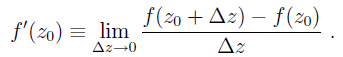Differentiation in the complex domain

So, the first challenge is how to make δz vanishingly small. In the complex plane, we can do it from multiple directions. As evident from the definition, the derivative of the function must not change in the way the limit is taken. Or else, we will get a new derivative every time we switch the limits. In fact, the thing we are doing is, investigating how the function f(z) changes when we take δz⇢0, or in other words, δx⇢0 and δy⇢0. In essence, our derivative must not change in changing the order of taking limits. And changing the order of limits doesn’t always guarantee the same value (every mathematician can cook up a function that proves this proposition!). So, differentiability is a non-trivial property in the complex world.

Now, let us write f(z)=u(z)+iv(z) or, equivalently, f(x,y)=u(x,y)+iv(x,y)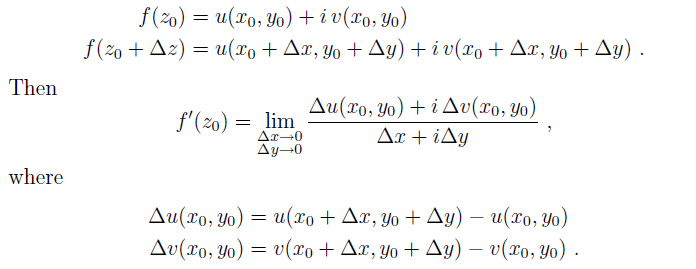Next, we take δx⇢0 first and then δy⇢0. This is equivalent to taking δz⇢0 along the imaginary axis.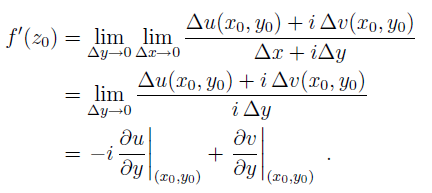Now, the opposite way, we take δy⇢0 first and then δx⇢0. This is equivalent to taking δz⇢0 along the real axis.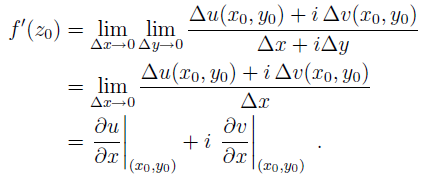The two expressions agree if and only if :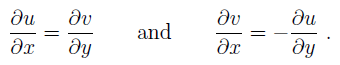Cauchy-Riemann equations

Here, we got the Cauchy-Riemann equations.

So, to check if a function f(z) is differentiable at a point zo=xo+iyo, we need to see if they satisfy the Cauchy-Riemann equations. And then their derivative is given by either expression obtained for f'(zo).

It can be observed that the differentiation in the complex world is indeed not easy and there are a lot of functions that aren’t really differentiable, even the most simple (try differentiating z*, the complex conjugate of z).

We can say a function is said to be analytic or holomorphic in a neighborhood of zo if it is differentiable in that neighborhood. A function that is analytic over the whole complex plane is said as entire.

It is important to realize that analyticity, unlike differentiability, is not a property of a function at a point, but on an open set of points. The reason for this is to be able to eliminate from the class of interesting functions, functions which may be differentiable at a point but nowhere else. Whereas this is a rarity in calculus, it is a very common occurrence for complex-valued functions of complex variables. For example, consider the function f(z) = |z|2. This function has u(x, y) = x2 + y2 and v(x, y) = 0. Therefore, the Cauchy–Riemann equations are only satisfied at the origin in the complex plane.

My Personal Notes arrow_drop_up
Recommended Articles
Page :